# Python数据分析之Numpy2018年4月26日06:53:121 2 6709字阅读22分21秒

## Numpy介绍

NumPy （Numerical Python 的简称），是高性能科学计算和数据分析的基础包。

NumPy 提供了对多维数组（矩阵）的高效支持，同时还有以下优点：

• ndarray, 核心数据结构，支持矢量运算的多维数组，在内存中进行连续存储；
• 各种操作多维数组和进行数组间运算的数学函数（不需要编写循环）；
• 用于集成其他编程语言的各种接口；

NumPy 本身没有提供高级的数据分析功能，但理解 NumPy 数组以及面向数组的各种计算有助于更加高效使用其他数据分析工具。

## 多维数组的创建

Numpy的核心是多维数组，通过`ndarray`对象做到，`ndarray`对象是一个灵活的数据容器，可以基于此数据容器对数据进行各种运算。

### 通过np.array创建数组

1. import numpy as np

``````a1 = np.array([[1,2,3,4],[5,6,7,8]])

a1.shape # 输出数组的形状
>> (2,4)

a1.ndim # 输出数组的维度,这里是二维数组
>> 2

a1.size # 输出数组中一共有多少元素,这里是4*2
>> 8

a1.max() # 输出a1中最大的元素
>> 8

a1
>> array([[1, 2, 3, 4],
[5, 6, 7, 8]])``````

### 其他创建数组的方法

• `np.arange`类似于 Python 内置的 range，创建一维数组；
• `np.ones`创建元素值全部为 1 的数组；
• `np.zeros`创建元素值全为 0 的数组；
• `np.empty`创建空值多维数组，只分配内存，不填充任何值；
• `np.random.random`创建元素值为随机值的多维数组；

#### np.arange创建数组

``````a1 = np.arange(1,4)
>> array([1,2,3])``````

#### np.ones创建数组

``````a1 = np.ones((4,4),dtype=np.int64)
>> array([[1, 1, 1, 1],
[1, 1, 1, 1],
[1, 1, 1, 1],
[1, 1, 1, 1]])``````

#### np.zeros创建数组

``````a1 = np.zeros((3,3))
>> array([[ 0.,  0.,  0.],
[ 0.,  0.,  0.],
[ 0.,  0.,  0.]])``````

#### np.random.random创建数组

``````a1 = 3*np.random.random((3,3))+3
>> array([[ 4.88904983,  5.16038279,  3.10275584],
[ 5.28394531,  5.50129641,  5.97119755],
[ 3.10108378,  3.4346571 ,  4.25520641]])``````

### 使用reshape创建数组

`reshape`方法并不能直接创建数组，而是可以将一个数组变成其他维度的数组。

### 多维变成一维

``````a1 = np.ones((2,2))

a1.reshape(4)
>> array([ 1.,  1.,  1.,  1.])

a1.reshape((1,4))
>> array([[ 1.,  1.,  1.,  1.]])``````

### 一维变成多维

``````a1 = np.arange(12)
a1.reshape((3,4))
>> array([[ 0,  1,  2,  3],
[ 4,  5,  6,  7],
[ 8,  9, 10, 11]])``````

## 多维数组索引

``````l = [1,2,3,4,5]

l[:2] #选择前两个元素
>> [1,2]

l[1:5:2]
>> [2,4]``````

### 一维数组切片

``````a1 = np.arange(7)

a1
>> array([0, 1, 2, 3, 4, 5, 6])

a1[:3] #取前三个数
>> array([0, 1, 2])

a1[0:6:2]
>> array([0,2,4])``````

### 多维数组切片

``````a1 = np.arange(12).reshape((3,4))
>> array([[ 0,  1,  2,  3],
[ 4,  5,  6,  7],
[ 8,  9, 10, 11]])

a1[1,1] #这个是选取第2行第2列的元素,因为下标是从0开始的
>> 5

a1 # 这个是选取第2行的元素a1
>> array([4, 5, 6, 7])

a1[:,1] # 这个是选取第1列的内容
>> array([1,5,9])``````

``````a1 = np.arange(12).reshape((2,2,3))
>> array([[[ 0,  1,  2],
[ 3,  4,  5]],

[[ 6,  7,  8],
[ 9, 10, 11]]])

a1[1,1,1]
>> 10

a1 # 注意和下面的进行比较
>> array([[ 6,  7,  8],
[ 9, 10, 11]])

a1[:,1] # 选取第二个维度的内容,可以理解为在第一个和第二个数组里的第二行
>> array([[ 3,  4,  5],
[ 9, 10, 11]])

a1[:,:,1] # 选取第三个维度的内容
>> array([[ 1,  4],
[ 7, 10]])``````

### numpy进行倒序输出

1. np.array([1,2,3,4,5,6])[::-1]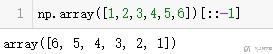## 数组的合并

### append 进行添加

1. a = np.array([1,2,3])
2. np.append(a, np.array([4,5]))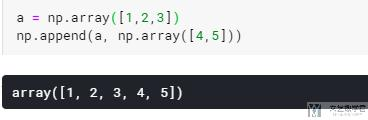### concatenate 进行合并

`numpy` 中,我们可以使用 `concatenate` 来进行合并：

1. a1 = np.array([1,2,3])
2. a2 = np.array([4,5,6])
3. # numpy进行合并
4. np.concatenate([a1, a2])
5. """
6. array([1, 2, 3, 4, 5, 6])
7. """

1. test = np.array([[1,2,3]])
2. np.concatenate((test, test))
3. """
4. array([[1, 2, 3],
5.        [1, 2, 3]])
6. """

1. a1 = [1,2,3]
2. a2 = [4,5,6]
3. # list进行合并
4. a1+a2
5. """
6. [1, 2, 3, 4, 5, 6]
7. """

### stack 进行合并

1. a = [
2.     np.array([1,2,3]),
3.     np.array([4,5,6]),
4.     np.array([7,8,9])
5. ]
6. np.stack(a)## numpy 中的数据类型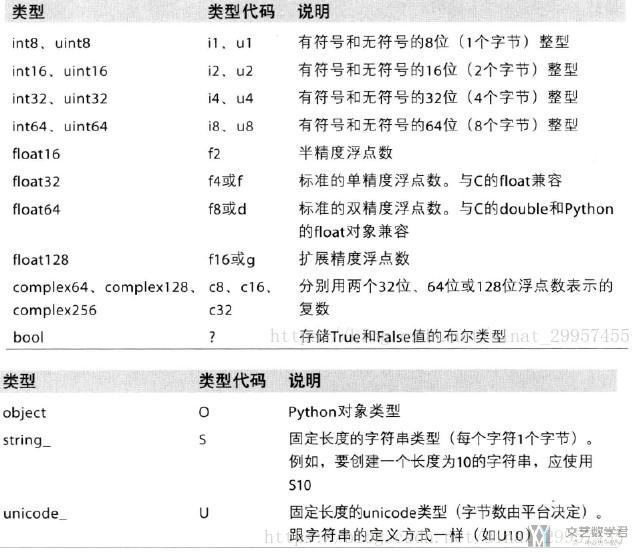## 数组的排序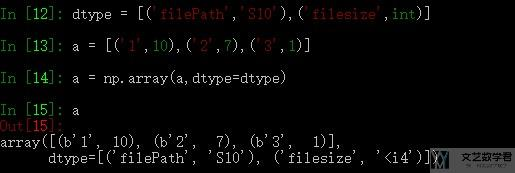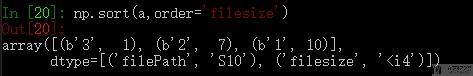## 多维数组的基础运算

``````l = [1,2,3]
[i+1 for i in l]
>> [2,3,4]``````

### 多维数组与标量的运算

``````a1 = np.arange(12).reshape((3,4))
>> array([[ 0,  1,  2,  3],
[ 4,  5,  6,  7],
[ 8,  9, 10, 11]])

a1+1
>> array([[ 1,  2,  3,  4],
[ 5,  6,  7,  8],
[ 9, 10, 11, 12]])

a1*2
>> array([[ 0,  2,  4,  6],
[ 8, 10, 12, 14],
[16, 18, 20, 22]])``````

### 多维数组间的运算

``````a1 = np.arange(1,5).reshape((2,2))
>> array([[1, 2],
[3, 4]])

a2 = np.arange(5,9).reshape((2,2))
>> array([[5, 6],
[7, 8]])

a1+a2
>> array([[ 6,  8],
[10, 12]])

a1*a2 # 这里不是矩阵乘法，是元素两两相乘
>> array([[ 5, 12],
[21, 32]])

a1.dot(a2) # 这个才是矩阵乘法
>> array([[19, 22],
[43, 50]])``````

## 多维数组的逻辑运算

``````a1 = np.random.random(9).reshape(3,3)
>> array([[ 0.92924529,  0.68050394,  0.09914842],
[ 0.5489492 ,  0.05738463,  0.35223837],
[ 0.43749942,  0.81850431,  0.75036973]])

b = a1 > .5
a1[b]
>> array([ 0.92924529,  0.68050394,  0.5489492 ,  0.81850431,  0.75036973])``````

## 一些统计方法

• a.sum 计算多维数组的所有元素的和；
• a.max 最大值计算；
• a.min 最小值计算；
• a.mean 平均值计算；
• a.std 标准差计算；
• a.var 方差计算；

• 指定`axis=0`时代表分别统计每列上的数据;
• 指定`axis=1`时，代表分别统计每一行上的数据;
• 没有指定`axis`参数时，代表统计所有元素。

1. a1 = np.arange(9).reshape((3,3))
2. >> array([[0, 1, 2],
3.        [3, 4, 5],
4.        [6, 7, 8]])
5. a1.var() # 计算方差
6. >> 6.66667
7. a1.std() # 计算标准差
8. >> 2.58198
9. a1.std()**2 #　标准差的平方为方差
10. >> 6.6667
11. a1.sum(axis=0) # 每一列求和
12. >> array([ 9, 12, 15])
13. a1.sum(axis=1) # 每一行求和
14. >> array([ 3, 12, 21])

### 每n个元素进行运算

1. """
2. Input:  np.array([1,2,3,1,2,3,1,2,3])
3. Output:  np.array([2,2,2])
4. """

1. np.mean(arr.reshape(-1, 3), axis=1)

### 找出数据中的指定值的位置

1. a = np.array(['a', 'b', 'c'])
2. np.where(a=='b')
3. """
4. (array(, dtype=int64),)
5. """

### 找出最大元素的位置

1. b = np.array([[1,2,30,5,3],[4,5,6,8,10]])
2. # 找出最大元素的值
3. b.max(axis=1)
4. """
5. array([30, 10])
6. """
7. # 找出最大元素的index
8. b.argmax(axis=1)
9. """
10. array([2, 4], dtype=int64)
11. """

### 统计每一个元素出现的次数

1. a = numpy.array([0, 3, 0, 1, 0, 1, 2, 1, 0, 0, 0, 0, 1, 3, 4])
2. unique, counts = numpy.unique(a, return_counts=True)
3. dict(zip(unique, counts))
4. # >>> {0: 7, 1: 4, 2: 1, 3: 2, 4: 1}

## 其他

### 交换axis

1. a = np.array([[[1,2],[5,6],[8,9]], [[1,2],[5,6],[8,9]],[[2,3],[5,6],[8,9]],[[2,3],[5,6],[8,9]]])
2. a.shape
3. """
4. (4, 3, 2)
5. """

1. b = np.transpose(a, (2,1,0))
2. b.shape
3. """
4. (2, 3, 4)
5. """

1. array([[[1, 1, 2, 2],
2.         [5, 5, 5, 5],
3.         [8, 8, 8, 8]],
4.        [[2, 2, 3, 3],
5.         [6, 6, 6, 6],
6.         [9, 9, 9, 9]]])

### 产生随机数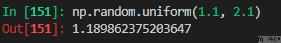### 关于取整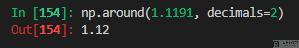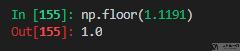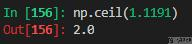### 设置显示精度

1. np.set_printoptions(suppress=True)
2. np.set_printoptions(precision=5) #设精度为5

• 微信公众号
• 关注微信公众号
•• QQ群
• 我们的QQ群号
•• 本文由 发表于 2018年4月26日06:53:12
• 转载请务必保留本文链接：https://mathpretty.com/9253.html

•zhanyu8008

很有帮助，赞